Students can download Maths Chapter 8 Statistics and Probability Additional Questions and Answers, Notes, Samacheer Kalvi 10th Maths Guide Pdf helps you to revise the complete Tamilnadu State Board New Syllabus, helps students complete homework assignments and to score high marks in board exams.

## Tamilnadu Samacheer Kalvi 10th Maths Solutions Chapter 8 Statistics and Probability Additional Questions

I. Multiple Choice Questions

Question 1.
The range of the first 10 prime numbers 2, 3, 5, 7, 11, 13, 17, 19, 23, 29 is ______
(1) 28
(2) 26
(3) 29
(4) 27
(4) 27
Hint:
Range = Largest value – Smallest value = 29 – 2 = 27

Question 2.
The least value in a collection of data is 14.1. If the range of the collection is 28.4, then the greatest value of the collection is _______
(1) 42.5
(2) 43.5
(3) 42.4
(4) 42.1
(1) 42.5
Hint:
Given, S = 14.1; R = 28.4,
L = S + R = 28.4 + 14.1 = 42.5Question 3.
The greatest value of a collection of data is 72 and the least value is 28. Then the coefficient of range is ______
(1) 44
(2) 0.72
(3) 0.44
(4) 0.28
(3) 0.44
Hint:
Coefficient of range = $$\frac{L-S}{L+S}$$
= $$\frac{72-28}{72+28}=\frac{44}{100}$$
= 0.44

Question 4.
For a collection of 11 items, Σx = 132, then the arithmetic mean is _______
(1) 11
(2) 12
(3) 14
(4) 13
(2) 12
Hint:
$$\bar{x}=\frac{\Sigma x}{n}=\frac{132}{11}=12$$

Question 5.
For any collection of n items, Σ(x – $$\bar{x}$$) = _____
(1) Σx
(2) $$\bar{x}$$
(3) n$$\bar{x}$$
(4) 0
(4) 0
Hint:
We know that, For all collection of n items,
Σ(x – $$\bar{x}$$) = 0

Question 6.
For any collection of n items, (Σx) – $$\bar{x}$$ = ________
(1) n$$\bar{x}$$
(2) (n – 2)$$\bar{x}$$
(3) (n – 1)$$\bar{x}$$
(4) 0
(3) (n – 1)$$\bar{x}$$
Hint:Question 7.
If t is the standard deviation of x, y, z, then the standard deviation of x + 5, y + 5, z + 5 is _______
(1) $$\frac{t}{3}$$
(2) t + 5
(3) t
(4) xyz
(3) t
Hint:
The S.D. of distribution remains unchanged when each value is added or subtracted by the same quantity.Question 8.
If the standard deviation of a set of data is 1.6, then the variance is _______
(1) 0.4
(2) 2.56
(3) 1.96
(4) 0.04
(2) 2.56
Hint:
Variance = (S.D.)2 = (1.6)2 = 2.56

Question 9.
If the variance of a data is 12.25, then the S.D is _______
(1) 3.5
(2) 3
(3) 2.5
(4) 3.25
(1) 3.5
Hint:
S.D = √Variance = √12.25 = 3.5

Question 10.
Variance of the first 11 natural numbers is ______
(1) √5
(2) √10
(3) 5√2
(4) 10
(4) 10
Hint:
Variance = $$\frac{n^{2}-1}{12}=\frac{11^{2}-1}{12}=\frac{120}{12}=10$$

Question 11.
The variance of 10, 10, 10, 10, 10 is _______
(1) 10
(2) √10
(3) 5
(4) 0
(4) 0
Hint:Question 12.
If the variance of 14, 18, 22, 26, 30 is 32, then the variance of 28, 36, 44, 52, 60 is _______
(1) 64
(2) 128
(3) 32√2
(4) 32
(2) 128
Hint:
Variance of the given numbers = 32;
S.D. = √32 = 4√2
Each data is multiplied by 2.
New S.D.= 4√2 × 2 = 8√2
Variance = (8√2)2 = 128Question 13.
The standard deviation of a collection of data is 2√2. If each value is multiplied by 3, then the standard deviation of the new data is ______
(1) √12
(2) 4√2
(3) 6√2
(4) 9√2
(3) 6√2
Hint:
Given, S.D. = 2√2
Each value is multiplied by 3
New S.D. = 2√2 × 3 = 6√2

Question 14.
Given Σ(x – $$\bar{x}$$)2 = 48, $$\bar{x}$$ = 20 and n = 12. The coefficient of variation is ______
(1) 25
(2) 20
(3) 30
(4) 10
(4) 10
Hint: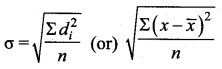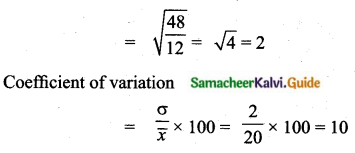Question 15.
Mean and standard deviation of a data are 48 and 12 respectively. The coefficient of variation is _______
(1) 42
(2) 25
(3) 28
(4) 48
(2) 25
Hint:
Coefficient of variation
C.V = $$\frac{\sigma}{\bar{x}} \times 100$$ = $$\frac{12}{48} \times 100$$ = 25

Question 16.
If Φ is an impossible event, then P(Φ) = ______
(1) 1
(2) $$\frac{1}{4}$$
(3) 0
(4) $$\frac{1}{2}$$
(3) 0
Hint:
Probability of an impossible event is 0.

Question 17.
If S is the sample space of a random experiment, then P(S) = _______
(1) 0
(2) $$\frac{1}{8}$$
(3) $$\frac{1}{2}$$
(4) 1
(4) 1
Hint:
Every event is a subset of S. Sample space contain all the possible element.
P(S) = 1

Question 18.
If p is the probability of an event A, then p satisfies _______
(1) 0 < p < 1
(2) 0 < p < 1
(3) 0 < p < 1
(4) 0 < p < 1
(2) 0 < p < 1
Hint:
The Probability of an event is always greater than 0 and less than or equal to 1.Question 19.
Let A and B be any two events and S be the corresponding sample space. Then P ($$\bar{A}$$ ∩ B) = ______(1) P(B) – P(A ∩ B)
(2) P(A ∩ B) – P(B)
(3) P(S)
(4) P[(A ∪ B)’]
(1) P(B) – P(A ∩ B)
Hint:
P($$\bar{A}$$ ∩ B) means only B and not A

Question 20.
The probability that a student will score centum in mathematics is $$\frac{4}{5}$$. The probability that he will not score centum is ______
(1) $$\frac{1}{5}$$
(2) $$\frac{2}{5}$$
(3) $$\frac{3}{5}$$
(4) $$\frac{4}{5}$$
(1) $$\frac{1}{5}$$
Hint:
P($$\bar{A}$$) = 1 – P(A) = 1 – $$\frac{4}{5}$$ = $$\frac{1}{5}$$

Question 21.
If A and B are two events such that P(A) = 0.25, P(B) = 0.05 and P(A ∩ B) = 0.14, then P(A ∪ B) = _____
(1) 0.61
(2) 0.16
(3) 0.14
(4) 0.6
(2) 0.16
Hint:
P(A ∪ B) = P(A) + P(B) – P(A ∩ B) = 0.25 + 0.05 – 0.14 = 0.16

Question 22.
There are 6 defective items in a sample of 20 items. One item is drawn at random. The that it is probability a non-defective item is ________
(1) $$\frac{1}{10}$$
(2) 0
(3) $$\frac{3}{10}$$
(4) $$\frac{2}{3}$$
(1) $$\frac{1}{10}$$
Hint:
Non-defective item = 20 – 6 = 14
Probability of non-defective items = $$\frac{14}{20}$$ = $$\frac{7}{10}$$

Question 23.
If A and B are mutually exclusive events and S is the sample space such that P(A) = $$\frac{1}{3}$$ P(B) and S = A ∪ B, then P(A) = _____
(1) $$\frac{1}{4}$$
(2) $$\frac{1}{2}$$
(3) $$\frac{3}{4}$$
(4) $$\frac{3}{8}$$
(1) $$\frac{1}{4}$$
Hint:
P(A) = $$\frac{1}{3}$$ P(B)
P(B) = 3 P(A)
P(A ∪ B) = P(A) + P(B)
[A and B are mutually exclusive]
P(A ∪ B) = P(A) + 3 P(A)
1 = 4P(A) [But P(A ∪ B) = 1]
P(A) = $$\frac{1}{4}$$

Question 24.
The probabilities of three mutually exclusive events A, B and C are given by $$\frac{1}{3}$$, $$\frac{1}{4}$$ and $$\frac{5}{12}$$. Then P(A ∪ B ∪ C) is _______
(1) $$\frac{19}{12}$$
(2) $$\frac{11}{12}$$
(3) $$\frac{7}{12}$$
(4) 1
(4) 1
Hint:
P (A ∪ B ∪ C) = P(A) + P(B) + P(C)
$$\frac{1}{3}+\frac{1}{4}=\frac{5}{12}=\frac{4+3+5}{12}=\frac{12}{12}=1$$Question 25.
If P(A) = 0.25, P(B) = 0.50, P(A ∩ B) = 0.14 then P(neither A nor B) = ______
(1) 0.39
(2) 0.25
(3) 0.11
(4) 0.24
(1) 0.39
Hint:
P(A ∪ B) = P(A) + P(B) – P(A ∩ B)
= 0.25 + 0.50 – 0.14
= 0.61
P (neither A nor B) = P($$\bar{A}$$ ∩ $$\bar{B}$$)
= 1 – P(A ∪ B)
= 1 – 0.61
= 0.39

Question 26.
A bag contains 5 black balls, 4 white balls and 3 red balls. If a ball is selected at random, the probability that it is not red is _______
(1) $$\frac{5}{12}$$
(2) $$\frac{4}{12}$$
(3) $$\frac{3}{12}$$
(4) $$\frac{3}{4}$$
(4) $$\frac{3}{4}$$
Hint:
P($$\bar{R}$$) = 1 – P(R)
$$=1-\frac{3}{12}=\frac{9}{12}=\frac{3}{4}$$

Question 27.
Two dice are thrown simultaneously. The probability of getting a doublet is ________
(1) $$\frac{1}{36}$$
(2) $$\frac{1}{3}$$
(3) $$\frac{1}{6}$$
(4) $$\frac{2}{3}$$
(3) $$\frac{1}{6}$$
Hint:
n(S) = 36
Let A be the event of getting a doublet
A = {(1, 1), (2, 2), (3, 3), (4, 4), (5, 5), (6, 6)}
n(A) = 6
P(A) = $$\frac{n(\mathrm{A})}{n(\mathrm{S})}=\frac{6}{36}=\frac{1}{6}$$

Question 28.
A fair die is thrown once. The probability of getting a prime or composite number is _______
(1) 1
(2) 0
(3) $$\frac{5}{6}$$
(4) $$\frac{1}{6}$$
(3) $$\frac{5}{6}$$
Hint:
S = {1, 2, 3, 4, 5, 6}
n(S) = 6
The required probability = $$\frac{5}{6}$$
[Science is neither prime not a composite number]

Question 29.
Probability of getting 3 heads or 3 tails in tossing a coin 3 times is _______
(1) $$\frac{1}{8}$$
(2) $$\frac{1}{4}$$
(3) $$\frac{3}{8}$$
(4) $$\frac{1}{2}$$
(2) $$\frac{1}{4}$$
Hint:
S= {HHH, HHT, HTH, THH, HTT, THT, TTH, TTT}
n(S) = 8
A = {HHH, TTT}; n(A) = 2
The required probability = $$\frac{2}{8}$$ = $$\frac{1}{4}$$

Question 30.
A card is drawn from a pack of 52 cards at random. The probability of getting neither an ace nor a king card is _____
(1) $$\frac{2}{13}$$
(2) $$\frac{11}{13}$$
(3) $$\frac{4}{13}$$
(4) $$\frac{8}{13}$$
(2) $$\frac{11}{13}$$
Hint:
n(S) = 52
Number of ace cards = 4
number of king cards = 4
n(non-ace and non-king cards) = 52 – 8 = 44
P(neither an ace nor a king) = $$\frac{44}{52}$$ = $$\frac{11}{13}$$Question 31.
The probability that a leap year will have 53 Fridays or 53 Saturdays is ______
(1) $$\frac{2}{7}$$
(2) $$\frac{1}{7}$$
(3) $$\frac{4}{7}$$
(4) $$\frac{3}{7}$$
(4) $$\frac{3}{7}$$
Hint:
Leap year contains 52 weeks and 2 days
Sample space = {(sun, mon), (mon, tue), (tue, wed), (wed, thu), (thu, fri), (fri, sat), (sat, sun)}
n(S) = 7
The required probability = $$\frac{2}{7}+\frac{2}{7}-\frac{1}{7}=\frac{3}{7}$$

Question 32.
The probability that a non-leap year will have 53 Sundays and 53 Mondays is ________
(1) $$\frac{1}{7}$$
(2) $$\frac{2}{7}$$
(3) $$\frac{3}{7}$$
(4) 0
(4) 0
Hint:
Non leap year contains 52 weeks and one day
Sample space (S) = {Sun, Mon, Tue, Wed, Thu, Fri, Sat}
n(S) = 7
The required probability = $$\frac{1}{7}+\frac{1}{7}-\frac{2}{7}$$
= $$\frac{2}{7}-\frac{2}{7}$$
= 0

Question 33.
The probability of selecting a queen of hearts when a card is drawn from a pack of 52 playing cards is ______
(1) $$\frac{1}{52}$$
(2) $$\frac{16}{52}$$
(3) $$\frac{1}{13}$$
(4) $$\frac{1}{26}$$
(1) $$\frac{1}{52}$$
Hint:
n(S) = 52 [1 queen of hearts in 52 cards]
n(A) = 1
P(A) = $$\frac{n(\mathrm{A})}{n(\mathrm{S})}=\frac{1}{52}$$

Question 34.
Probability of sure event is ______
(1) 1
(2) 0
(3) 100
(4) 0.1
(1) 1

Question 35.
The outcome of a random experiment result in either success or failure. If the probability of success is twice the probability of failure, then the probability of success is ______
(1) $$\frac{1}{3}$$
(2) $$\frac{2}{3}$$
(3) 1
(4) 0
(2) $$\frac{2}{3}$$
Hint:
n(A ∪ B) = 1; P($$\bar{A}$$) = 1 – P(A)
Given P(A) = 2P($$\bar{A}$$)
P(A) = 2 [1 – P(A)] = 2 – 2 P(A)
3 P(A) = 2
P(A) = $$\frac{2}{3}$$

Question 1.
Find the range and the coefficient of range of the following data.Largest value (L) = 690
Smallest value (S) = 610
Range R = L – S = 690 – 610 = 80
Coefficient of range = $$\frac{L-S}{L+S}=\frac{690-610}{690+610}=\frac{80}{1300}$$ = 0. 06

Question 2.
Two dice are thrown simultaneously. What is the probability that
(i) 5 will not come up on either of them
(ii) 5 will come up at both dice
S = {(1, 1)(1, 2)(1, 3)(1, 4)(1, 5)(1, 6), (2, 1)(2, 2)(2, 3)(2, 4)(2, 5)(2, 6), (3, 1)(3, 2)(3, 3)(3, 4)(3, 5)(3, 6), (4, 1)(4, 2)(4, 3)(4, 4)(4, 5)(4, 6), (5, 1)(5, 2)(5, 3)(5, 4)(5, 5)(5, 6), (6, 1)(6, 2)(6, 3)(6, 4)(6, 5)(6, 6)}
n(S) = 36
(i) Let A be the event of getting 5 on either of them.
A = {(1, 5) (2, 5) (3, 5) (4, 5) (5, 1) (5, 2) (5, 3) (5, 4) (5, 5) (5, 6) (6, 5)}
n(A) = 11
P(A) = $$\frac{n(\mathrm{A})}{n(\mathrm{S})}=\frac{11}{36}$$
Probability that 5 will not come up on either of them = 1 – P (A)
$$=1-\frac{11}{36}=\frac{36-11}{36}=\frac{25}{36}$$
(ii) Let B be the event of getting 5 will come up at both dice
B = {(5, 5)}
n(B) = 1
P(B) = $$\frac{n(\mathrm{B})}{n(\mathrm{S})}=\frac{1}{36}$$

Question 3.
The king, Queen and Jack of clubs are removed from a deck of 52 playing cards and the remaining cards are shuffled. A card is drawn from the remaining cards, find the probability of getting
(i) a card of clubs
(ii) a queen of diamond
Sample space (S) = (52 – 3) = 49
n (S) = 49
(i) Let A be the event of getting a card of clubs.
n(A) = (13 – 3) = 10
P(A) = $$\frac{n(\mathrm{A})}{n(\mathrm{S})}=\frac{10}{49}$$
(ii) Let B be the event of getting a queen of diamond
n(B) = 1
P(B) = $$\frac{n(\mathrm{B})}{n(\mathrm{S})}=\frac{1}{49}$$Question 4.
The standard deviation of 20 observations is √5. If each observation is multiplied by 2, find the standard deviation and variance of the resulting observations.
Given a standard deviation of 20 observations = √5
Each observation is multiplied by 2 then,
New standard deviation = 2 × √5 = 2√5
New variance = (2√5)2 = 20

Question 5.
Calculate the variance standard deviation of the following data 38, 40, 34, 31, 28, 26, 34.
Arrange the given data in ascending order we get, 26, 28, 31, 34, 38, 40
Assumed mean = 34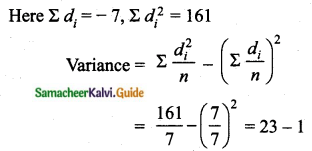Variance = 22
Standard deviation(σ) = √Variance = √22 = 4.69

Question 6.
Mean of 100 items is 48 and their standard deviation is 10. Find the sum of all the times and the sum of the squares of all items.
The mean of 100 items = 48
The sum of 100 items (Σx) = 100 × 48 = 4800
standard deviation (σ2) = 10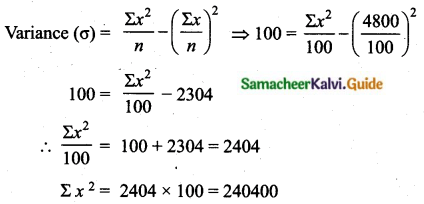Sum of the squares of all items (Σx2) = 240400

Question 7.
If n = 10, $$\bar{x}$$ = 12 and Σx2 = 1530, then calculate the coefficient of variation.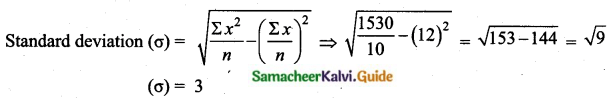Coefficient of variation = $$\frac{\sigma}{\bar{x}} \times 100 \Rightarrow \frac{3}{12} \times 100=25$$

Question 8.
If the coefficient of variation of a collection of data is 57 and its standard deviation is 6, 84, then find the mean.
Given the coefficient of variation = 57
Standard deviation (σ) = 6.84Arithmetic mean = $$\bar{x}$$ = 12

Question 9.
Find the standard deviation and the variance of first 23 natural numbers?
Standard deviation of first “n” natural numbers = $$\sqrt{\frac{n^{2}-1}{12}}$$
Standard deviation of first “23” natural numbers = $$\sqrt{\frac{23^{2}-1}{12}}$$
$$=\sqrt{\frac{529-1}{12}}=\sqrt{\frac{528}{12}}=\sqrt{44}$$
= 6.63

Question 10.
Find the coefficient of variation of the following data: 18, 20, 15, 12, 25.
Let us calculate the A.M of the given data.The coefficient of variation is 24.6

Question 11.
Three rotten eggs are mixed with 12 good ones. One egg is chosen at random. What is the probability of choosing a rotten egg?
Number of good eggs = 12
Number of rotton eggs = 3
Total number of eggs = 12 + 3 = 15
Sample space n(S) = 15
Let A be the event of choosing a rotten egg
n(A) = 3
P(A) = $$\frac{n(\mathrm{A})}{n(\mathrm{S})}=\frac{3}{15}=\frac{1}{5}$$
The Probability is $$\frac{1}{5}$$Question 12.
Two coins are tossed together. What is the probability of getting at most one head?
Sample space (S) = {(H, H), (H, T), (T, H), (T, T)}
n(S) = 4
Let A be the event of getting atmost one head
A = {HT, TH, TT}
n(A) = 3
P(A) = $$\frac{n(\mathrm{A})}{n(\mathrm{S})}=\frac{3}{4}$$
The probability is $$\frac{3}{4}$$

Question 13.
A number is selected at random from integers 1 to 100. Find the probability that it is
(i) a perfect square
(ii) not a perfect cube.
Sample space (S) = {1, 2, 3, …,100}
n(S) = 100
(i) Let A be the event of getting a perfect square.
A = {1, 4, 9, 16, 25, 36, 49, 64, 81, 100}
n(A) = 10
P(A) = $$\frac{n(\mathrm{A})}{n(\mathrm{S})}=\frac{10}{100}=\frac{1}{10}$$
(ii) Let B be the event of getting a perfect cube.
B = {1, 8, 27, 64}
n(B) = 4
P(B) = $$\frac{n(\mathrm{B})}{n(\mathrm{S})}=\frac{4}{100}=\frac{1}{25}$$
The Probability that the selected number is not a perfect cube is
P($$\bar{B}$$) = 1 – P(B)
P($$\bar{B}$$) = 1 – $$\frac{1}{25}$$ = $$\frac{24}{25}$$

Question 14.
Three dice are thrown simultaneously. Find the probability of getting the same number on all the three dice.
Sample space (S) = {(1, 1, 1) (1, 1, 2) (1, 1, 3) ….(6, 6, 6)}
n(S) = 63 = 216
Let A be the event of getting the same number on all the three dice.
A = {(1, 1, 1) (2, 2, 2) (3, 3, 3) (4, 4, 4) (5, 5, 5) (6, 6, 6)}
n(A) = 6
P(A) = $$\frac{n(A)}{n(S)}=\frac{6}{216}=\frac{1}{36}$$
The probability is $$\frac{1}{36}$$

Question 15.
If P(A) = $$\frac{1}{2}$$, P(B) = $$\frac{7}{10}$$, P(A∪B) = 1, Find
(i) P(A ∩ B)
(ii) P(A’ ∪ B’)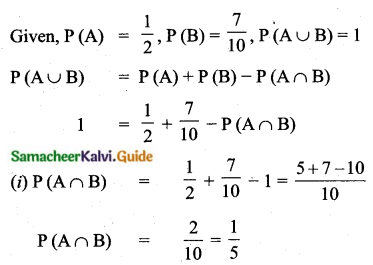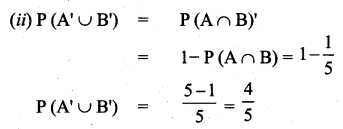Question 1.
The mean of the following frequency distribution is 53 and the sum of all frequencies is 100. compute the missing frequencies f1 and f2.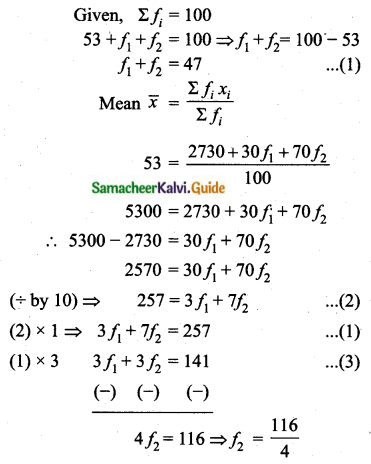f2 = 29
Substitute the value of f2 = 29 in (1)
f1 + 29 = 47
⇒ f1 = 47 – 29 = 18
The value of f1 = 18 and f2 = 29

Question 2.
Calculate the standard deviation of the following data.Assumed mean (A) = 13Standard deviation = 6.32

Question 3.
The time (in seconds) taken by a group to walk across a pedestrian crossing is given in the table below.Calculate the variance and standard deviation of the data.
Assumed mean (A) = 17.5, c = 5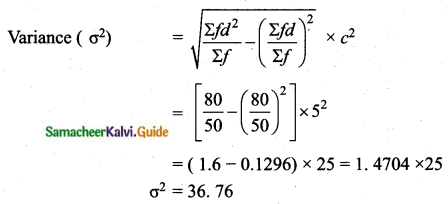Standard deviation (σ) = √36.76 = 6.063
Variance 36. 76, Standard deviation = 6.06

Question 4.
The mean and standard deviation of 20 items are found to be 10 and 2 respectively. At the time of checking it was found that an item 12 was wrongly entered as 8. Calculate the correct mean and standard deviation.
Given, mean of 20 items ($$\bar{x}$$) = 10
and standard deviation (σ) = 2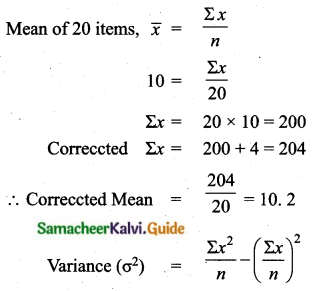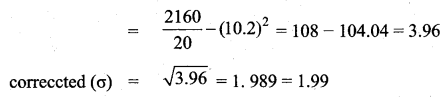(i) Corrected mean = 10. 2
(ii) Corrected Standard deviaton = 1.99Question 5.
Calculate the coefficient of variation of the following data: 20, 18, 32, 24, 26.
Arrange in ascending order, we get 18, 20, 24, 26, 32
Assumed mean = 24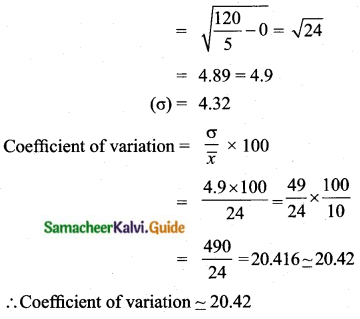Question 6.
The marks scored by two students A, B in a class are given below.Who is more consistent?
Student = A
$$\bar{x}$$ = 60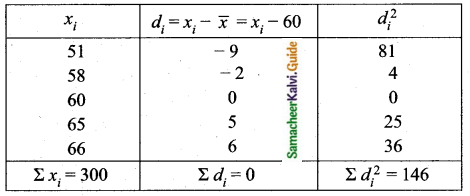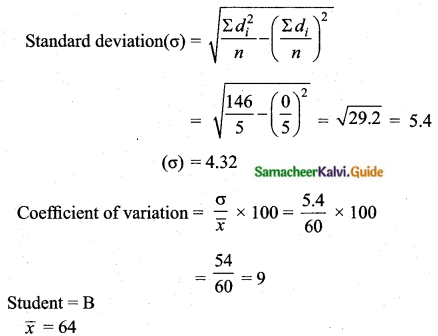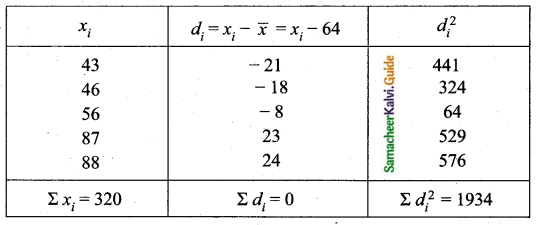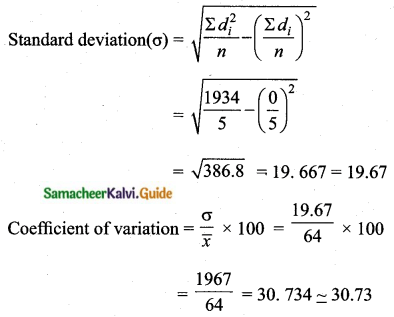From student A and B the coefficient of variation for A is less than the coefficient of variation for B.
Student A is more Consistent.

Question 7.
If for distribution Σ(x – 7) = 3, Σ(x – 7)2 = 57 and total number of item is 20. find the mean and standard deviation.(i) Arithmetic mean = 7. 15
(ii) Standard deviation = 1.68

Question 8.
Two unbiased dice are rolled once. Find the probability of getting
(i) a sum 8
(ii) a doublet
(iii) a sum greater than 8
When two dice are thrown, the sample space is
Sample space = {(1, 1), (1, 2), (1, 3), (1, 4), (1, 5), (1, 6), (2, 1), (2, 2), (2, 3), (2, 4), (2, 5), (2, 6), (3, 1), (3, 2), (3, 3), (3, 4), (3, 5), (3, 6), (4, 1), (4, 2), (4, 3), (4, 4), (4, 5), (4, 6), (5, 1), (5, 2), (5, 3), (5, 4), (5, 5), (5, 6), (6, 1), (6, 2), (6, 3), (6, 4), (6, 5), (6, 6)}
n(S) = 6 × 6 = 36
(i) Let A be the event of getting a sum 8
A= {(2, 6) (3, 5) (4, 4) (5, 3) (6, 2)}
n(A) = 5
P(A) = $$\frac{n(\mathrm{A})}{n(\mathrm{S})}=\frac{5}{36}$$
(ii) Let B be the event of getting a doublet.
B = {(1, 1) (2, 2) (3, 3) (4, 4) (5, 5) (6, 6)}
n(B) = 6
P(B) = $$\frac{n(\mathrm{B})}{n(\mathrm{S})}=\frac{6}{36}=\frac{1}{6}$$
(iii) Let C be the event of getting a sum greater than 8
C = {(3, 6) (4, 5) (4, 6) (5, 4) (5, 5) (5, 6) (6, 3) (6, 4) (6, 5) (6, 6)}
n(C) = 10
P(C) = $$\frac{n(\mathrm{C})}{n(\mathrm{S})}=\frac{10}{36}=\frac{5}{18}$$

Question 9.
A die is thrown twice. Find the probability that atleast one of the two throws conies up with the number 5 (use addition theorem).
In rolling a die twice, the size of the sample space, n(S) = 36
Let A be the event of getting 5 in the first throw.
A = {(5, 1) (5, 2) (5, 3) (5, 4) (5, 5) (5, 6)}
Thus, n(A) = 6, and P(A) = $$\frac{n(\mathrm{A})}{n(\mathrm{S})}=\frac{6}{36}$$
Let B be the event of getting 5 in the second throw.
B = {(1, 5) (2, 5) (3, 5) (4, 5) (5, 5) (6, 5)}
Thus, n(B) = 6, and P(B) = $$\frac{n(\mathrm{B})}{n(\mathrm{S})}=\frac{6}{36}$$
A and B are not mutually exclusive events, since A ∩ B = {(5, 5)}
n(A ∩ B) = 1 and P(A ∩ B) = $$\frac{1}{36}$$
P(A ∪ B) = P(A) + P(B) – P(A ∩ B)
$$=\frac{6}{36}+\frac{6}{36}-\frac{1}{36}=\frac{11}{36}$$

Question 10.
Let A, B, C be any three mutually exclusive and exhaustive events such that P(B) = $$\frac{3}{2}$$ P(A) and P(C) = $$\frac{1}{2}$$ P(B). Find P(A).
Let P(A) = p
Now, P(B) = $$\frac{3}{2}$$, P(A) = $$\frac{3}{2}$$ p
Also, P(C) = $$\frac{1}{2}$$ P(B)
= $$\frac{1}{2}$$ ($$\frac{3}{2}$$ p) = ($$\frac{3}{4}$$ p)
Given that A, B and C are mutually exclusive and exhaustive events.
P(A ∪ B ∪ C) = P(A) + P(B) + P(C) and S = A ∪ B ∪ C
Now, P(S) = 1
That is, P(A) + P(B) + P(C) = 1
p + $$\frac{3}{2}$$ p + $$\frac{3}{4}$$ p = 1
⇒ 4p + 6p + 3p = 4
Thus, p = $$\frac{4}{13}$$
Hence, P(A) = $$\frac{4}{13}$$Question 11.
A bag contains 50 bolts and 150 nuts. Half of the bolts and half of the nuts are rusted. If an item is chosen at random, find the probability that it is rusted or that it is a bolt.
Sample space (S) = (50 + 150) = 200
n(S) = 200
Number of rusted bolts = $$\frac{1}{2}$$ (50) = 25
Number of rusted nuts = $$\frac{1}{2}$$ (150) = 75
Let A be the event of getting rusted bolts and nuts.
n (A) = 25 + 75 = 100
P(A) = $$\frac{n(\mathrm{A})}{n(\mathrm{S})}=\frac{100}{200}$$
Let B be the event of getting a bolt.
n(B) = 50
P(B) = $$\frac{n(\mathrm{B})}{n(\mathrm{S})}=\frac{50}{200}$$
Number of bolts which are rusted n(A ∩ B) = 25Question 12.
Two dice are rolled simultaneously. Find the probability that that sum of the numbers on the faces is neither divisible by 3 nor by 4.
Sample space = {(1, 1), (1, 2), (1, 3), (1, 4), (1, 5), (1, 6), (2, 1), (2, 2), (2, 3), (2, 4), (2, 5), (2, 6), (3, 1), (3, 2), (3, 3), (3, 4), (3, 5), (3, 6), (4, 1), (4, 2), (4, 3), (4, 4), (4, 5), (4, 6), (5, 1), (5, 2), (5, 3), (5, 4), (5, 5), (5, 6), (6, 1), (6, 2), (6, 3), (6, 4), (6, 5), (6, 6)}
n(S) = 36
Let A be the event of getting the sum is divisible by 3
A = {(1, 2) (2, 1) (1, 5) (5, 1) (2, 4) (4, 2) (3, 3) (3, 6) (6, 3) (4, 5) (5, 4) (6, 6)}
n(A) = 12
P(A) = $$\frac{n(\mathrm{A})}{n(\mathrm{S})}=\frac{12}{36}$$
Let B be the event of getting a sum is divisible by 4.
B = {(1, 3) (2, 2) (2, 6) (3, 1) (3, 5) (4, 4) (5, 3) (6, 2) (6, 6)}
n(B) = 9
P(B) = $$\frac{n(\mathrm{B})}{n(\mathrm{S})}=\frac{9}{36}$$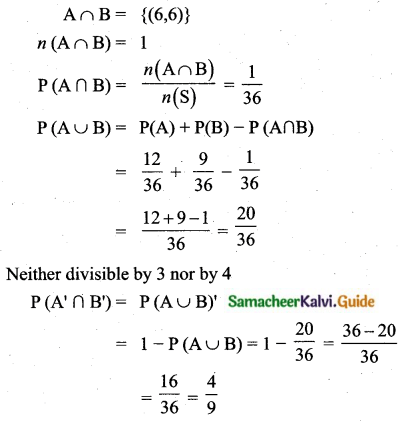Question 13.
In a class, 40% of the students participated in Mathematics-quiz, 30% in Science -quiz and 10% in both the quiz programmes. If a students is selected at random from the class, find the probability that the students participated in Mathematics or science or both quiz programmes.
Sample space (S) = 100
n(S) = 100
Let A be the event of getting a number of students participated in mathematics-quiz programme
n(A) = 40
P(A) = $$\frac{n(\mathrm{A})}{n(\mathrm{S})}=\frac{40}{100}$$
Let B be the event getting a number of students participated in science-quiz programme
n(B) = 30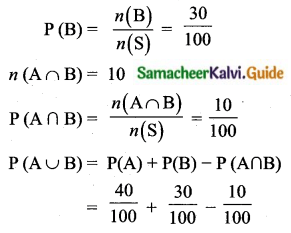$$=\frac{40+30-10}{100}=\frac{60}{100}=\frac{3}{5}$$
The required probability = $$\frac{3}{5}$$

Question 14.
A two digit number is formed with the digits 2, 5, 9 (repetition is allowed). Find the probability that the number is divisible by 2 or 5.
Sample space (S) = {22, 25, 29, 55, 52, 59, 99, 92, 95}
n(S) = 9
Let A be the event of getting number is divisible by 2.
A = {22, 52, 92}
n(A) = 3
P(A) = $$\frac{n(\mathrm{A})}{n(\mathrm{S})}=\frac{3}{9}$$
Let B be the event of getting number is divisible by 5.
B = {25, 95, 55}
n(B) = 3
P(B) = $$\frac{n(\mathrm{B})}{n(\mathrm{S})}=\frac{3}{9}$$
If A and B are mutually exclusive.
P(A ∪ B) = P(A) + P(B)
P(A ∪ B) = $$\frac{3}{9}+\frac{3}{9}=\frac{6}{9}=\frac{2}{3}$$
The required probability is $$\frac{2}{3}$$

Question 15.
The probability that A, B and C can solve a problem are $$\frac{4}{5}$$, $$\frac{2}{3}$$ and $$\frac{3}{7}$$ respectively. The probability of the problem being solved by A and B is $$\frac{8}{15}$$, B and C is $$\frac{2}{7}$$, A and C is $$\frac{12}{35}$$. The probability of the problem being solved by all the three is $$\frac{8}{35}$$. Find the probability that the problem can be solved by atleast one of them.
Given P(A) = $$\frac{4}{5}$$
P(B) = $$\frac{2}{3}$$
P(C) = $$\frac{3}{7}$$
P(A ∩ B) = $$\frac{8}{15}$$
P(B ∩ C) = $$\frac{2}{7}$$
P(A ∩ C) = $$\frac{12}{35}$$
P(A ∩ B ∩ c) = $$\frac{8}{35}$$The probability of the problem can be solved by at least one of them = $$\frac{101}{105}$$